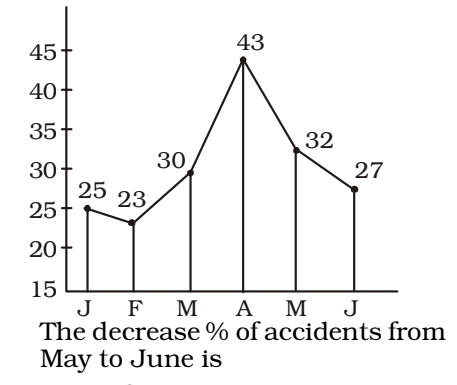## Introduction to Data Interpretation

#### Data Interpretation

Direction: Study the following graph and answer the questions.1. In 2005, the exports of company X were double that of company Y in that year. If the imports of company X during the year were \$ 180 million, what was the amount (in million) of imports of company Y during the year?

1. From the above given graph ,
In the year 2005, Imports of company X = \$ 180 million
Exports = 1.75 × 180 = \$ 315 million
Exports of company Y = \$ 157.5 million

 ∴ Imports of company Y = 157.5 0.75

##### Correct Option: B

From the above given graph ,
In the year 2005, Imports of company X = \$ 180 million
Exports = 1.75 × 180 = \$ 315 million
Exports of company Y = \$ 157.5 million

 ∴ Imports of company Y = 157.5 0.75

∴ Imports of company Y = \$ 210 million

1. If the imports of company X in 2007 were increased by 40%, what would be the ratio of exports to the increased imports?

1. On the basis of given graph in question ,

 Exports = 1.75 Imports

 = 175 = 7 100 4

##### Correct Option: A

On the basis of given graph in question ,

 Exports = 1.75 Imports

 = 175 = 7 100 4

 After 40% increase in imports, Exports = 7 = 700 Imports (4 × 140) / 100 4 × 140

 Required ratio = 5 = 1.25 4

1. Given is a line graph showing the number of accidents in a city during the first 6 months of 1999.1. According to given graph in question ,

 Percentage decrease = 32 - 27 × 100 32

##### Correct Option: C

According to given graph in question ,

 Percentage decrease = 32 - 27 × 100 32

 Percentage decrease = 5 × 100 = 125 = 15 5 % 32 8 8

1. The adjoining diagram is frequency polygon for the scores of students in a test. What is the total number of students appeared in the test?1. As per the given graph in question ,
Number of students = 15 + 30 + 35 + 30 + 25 + 22.5 + 22.5 = 180

##### Correct Option: A

As per the given graph in question ,
Number of students = 15 + 30 + 35 + 30 + 25 + 22.5 + 22.5 = 180
Hence , the total number of students appeared in the test is 180 .

Direction: Study the following graph and answer the questions.1. If the income shows positive growth every year throughout the period (2000 - 2005), then in how many years the expenditure shows a positive growth?

1. It is obvious from the graph.

##### Correct Option: D

It is obvious from the graph.
Hence , required answer is 2 .